# Module "Equations"

September 13, 2023

# 1General information about the editor

Just like figures and tables, formulas can now be entered directly in SMASHDOCs and displayed in a graphical view. The KaTeX editor used for this purpose is based on the TeX markup language, which can be entered and edited directly in SMASHDOCs.

Word documents that already contain (real) formulas are also represented in this notation.

For users who work intensively with the formula editor in SMASHDOCs, it is recommended to use the (very technical) online documentation of the tool under:

For getting started with creating and editing formulas, the interface built into SMASHDOCs and described below should provide adequate information.

# 2Insert an equation

A user can create various equations by clicking on the "Insert Formula" tab in the formatting bar.The "Insert formula" tab highlighted in the formatting bar

The new modal dialogue for creating an equation will be displayed. The user enters the equation with appropriate symbols and sees the final version in the "Formula Preview" window. Additionally, the description and/or automatic numbering can be provided.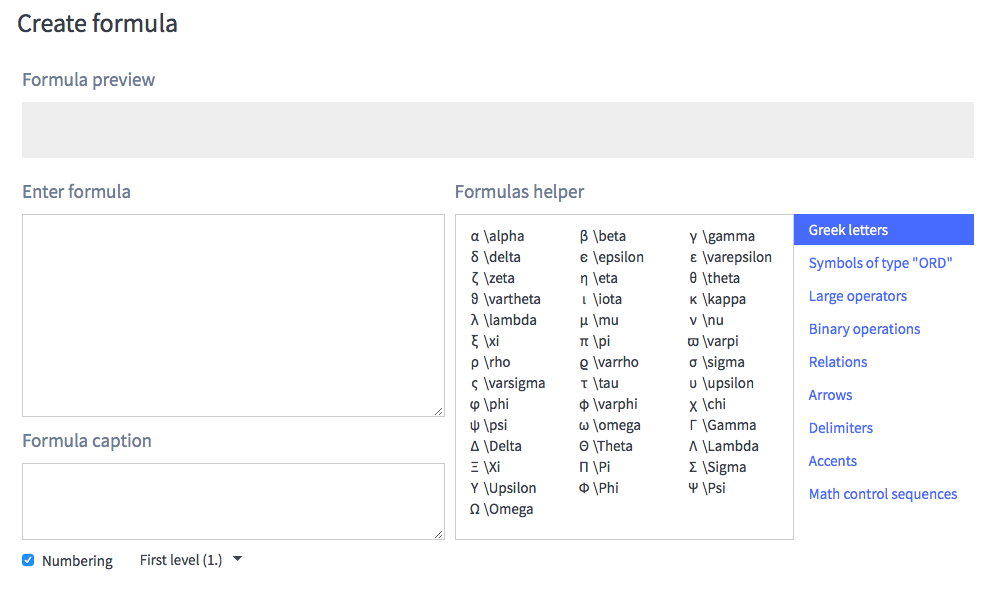Modal dialogue "Create Formula" highlighted

The user can also get a help to enter an equation correctly. For this, the user should proceed to the "Formulas helper" and choose between numerous symbols, operations, relations, etc.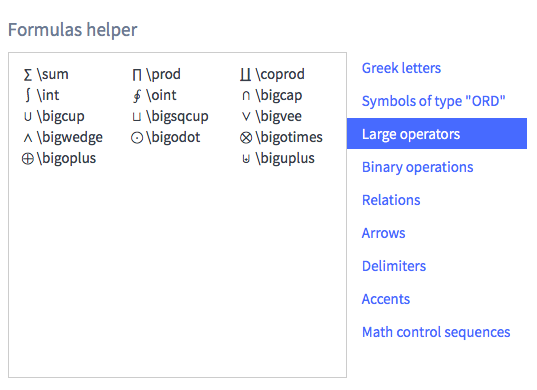The "Formulas helper" area highlighted in the "Create formula" modal dialogue

Using the window "Formulas helper", the user enters the equation in the "Enter formula" window and sees if his formula has been entered correctly in the "Formula preview" window.

If an error occurs, the editor will not allow the creation of an incorrect formula. The error type will be displayed in the "Formula preview" window.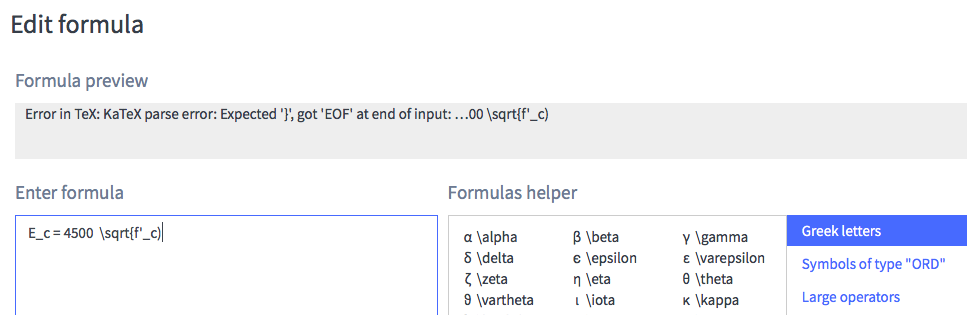Error after entering an incorrect equation highlighted in the "Formula Preview" line

Here are some formulas examples: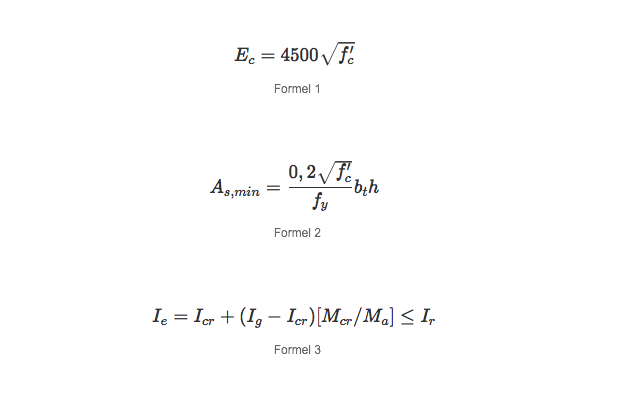Formulas examples

After opening the context menu of an equation, a user clicks on the "Edit formula" in order to manage it. Only users with "Suggest" and "Approver" roles can edit the formula. All changes are logged in review mode.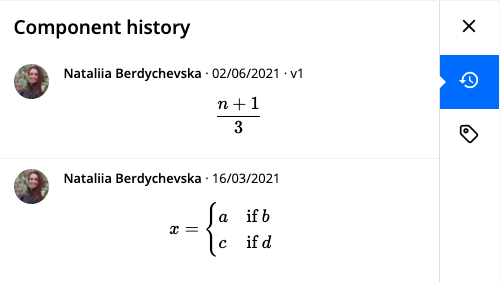Changed formula highlighted in the component history

The SD documents with inserted formulas can be exported to Word, sdox file, ParsX XML file and Annotation Report.

# 3Insert an inline equation

Inline formula can be inserted in text or table component.

To create the inline formula in the text component, click somewhere inside of the text component and click on the "Inline formula" tab in the formatting bar.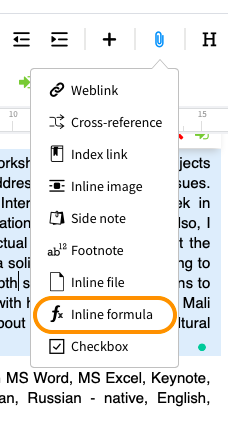The "Insert formula" tab highlighted in the formatting bar

The process of creating the inline formula is the same as described above for the standard formula.

To insert the inline formula in the table, click twice in the table cell and click on the "Inline formula" icon in the cells formatting bar.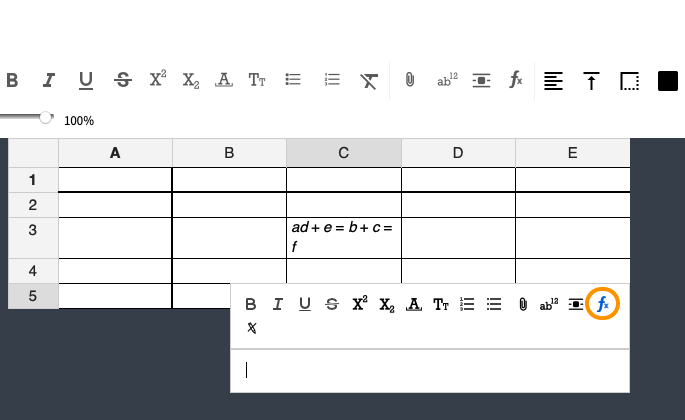Icon for "Insert formula" highlighted in the cells table bar​

Here`s an example of how inline formula looks in the document: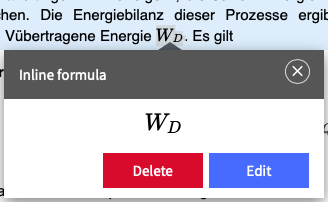Inline formula highlighted in the document